Perform a 2D kernel density estimation using MASS::kde2d() and display the results with contours. This can be useful for dealing with overplotting. This is a 2D version of geom_density(). geom_density_2d() draws contour lines, and geom_density_2d_filled() draws filled contour bands.

geom_density_2d(
mapping = NULL,
data = NULL,
stat = "density_2d",
position = "identity",
...,
contour_var = "density",
lineend = "butt",
linejoin = "round",
linemitre = 10,
na.rm = FALSE,
show.legend = NA,
inherit.aes = TRUE
)

geom_density_2d_filled(
mapping = NULL,
data = NULL,
stat = "density_2d_filled",
position = "identity",
...,
contour_var = "density",
na.rm = FALSE,
show.legend = NA,
inherit.aes = TRUE
)

stat_density_2d(
mapping = NULL,
data = NULL,
geom = "density_2d",
position = "identity",
...,
contour = TRUE,
contour_var = "density",
n = 100,
h = NULL,
na.rm = FALSE,
show.legend = NA,
inherit.aes = TRUE
)

stat_density_2d_filled(
mapping = NULL,
data = NULL,
geom = "density_2d_filled",
position = "identity",
...,
contour = TRUE,
contour_var = "density",
n = 100,
h = NULL,
na.rm = FALSE,
show.legend = NA,
inherit.aes = TRUE
)

## Arguments

mapping Set of aesthetic mappings created by aes() or aes_(). If specified and inherit.aes = TRUE (the default), it is combined with the default mapping at the top level of the plot. You must supply mapping if there is no plot mapping. The data to be displayed in this layer. There are three options: If NULL, the default, the data is inherited from the plot data as specified in the call to ggplot(). A data.frame, or other object, will override the plot data. All objects will be fortified to produce a data frame. See fortify() for which variables will be created. A function will be called with a single argument, the plot data. The return value must be a data.frame, and will be used as the layer data. A function can be created from a formula (e.g. ~ head(.x, 10)). Position adjustment, either as a string, or the result of a call to a position adjustment function. Arguments passed on to geom_contour binsNumber of contour bins. Overridden by binwidth. binwidthThe width of the contour bins. Overridden by breaks. breaksNumeric vector to set the contour breaks. Overrides binwidth and bins. By default, this is a vector of length ten with pretty() breaks. Character string identifying the variable to contour by. Can be one of "density", "ndensity", or "count". See the section on computed variables for details. Line end style (round, butt, square). Line join style (round, mitre, bevel). Line mitre limit (number greater than 1). If FALSE, the default, missing values are removed with a warning. If TRUE, missing values are silently removed. logical. Should this layer be included in the legends? NA, the default, includes if any aesthetics are mapped. FALSE never includes, and TRUE always includes. It can also be a named logical vector to finely select the aesthetics to display. If FALSE, overrides the default aesthetics, rather than combining with them. This is most useful for helper functions that define both data and aesthetics and shouldn't inherit behaviour from the default plot specification, e.g. borders(). Use to override the default connection between geom_density_2d() and stat_density_2d(). If TRUE, contour the results of the 2d density estimation. Number of grid points in each direction. Bandwidth (vector of length two). If NULL, estimated using MASS::bandwidth.nrd(). A multiplicative bandwidth adjustment to be used if 'h' is 'NULL'. This makes it possible to adjust the bandwidth while still using the a bandwidth estimator. For example, adjust = 1/2 means use half of the default bandwidth.

## Aesthetics

geom_density_2d() understands the following aesthetics (required aesthetics are in bold):

• x

• y

• alpha

• colour

• group

• linetype

• size

Learn more about setting these aesthetics in vignette("ggplot2-specs").

geom_density_2d_filled() understands the following aesthetics (required aesthetics are in bold):

• x

• y

• alpha

• colour

• fill

• group

• linetype

• size

• subgroup

Learn more about setting these aesthetics in vignette("ggplot2-specs").

## Computed variables

stat_density_2d() and stat_density_2d_filled() compute different variables depending on whether contouring is turned on or off. With contouring off (contour = FALSE), both stats behave the same, and the following variables are provided:

density

The density estimate.

ndensity

Density estimate, scaled to a maximum of 1.

count

Density estimate * number of observations in group.

n

Number of observations in each group.

With contouring on (contour = TRUE), either stat_contour() or stat_contour_filled() (for contour lines or contour bands, respectively) is run after the density estimate has been obtained, and the computed variables are determined by these stats. Contours are calculated for one of the three types of density estimates obtained before contouring, density, ndensity, and count. Which of those should be used is determined by the contour_var parameter.

geom_contour(), geom_contour_filled() for information about how contours are drawn; geom_bin2d() for another way of dealing with overplotting.

## Examples

m <- ggplot(faithful, aes(x = eruptions, y = waiting)) +
geom_point() +
xlim(0.5, 6) +
ylim(40, 110)

# contour lines
m + geom_density_2d()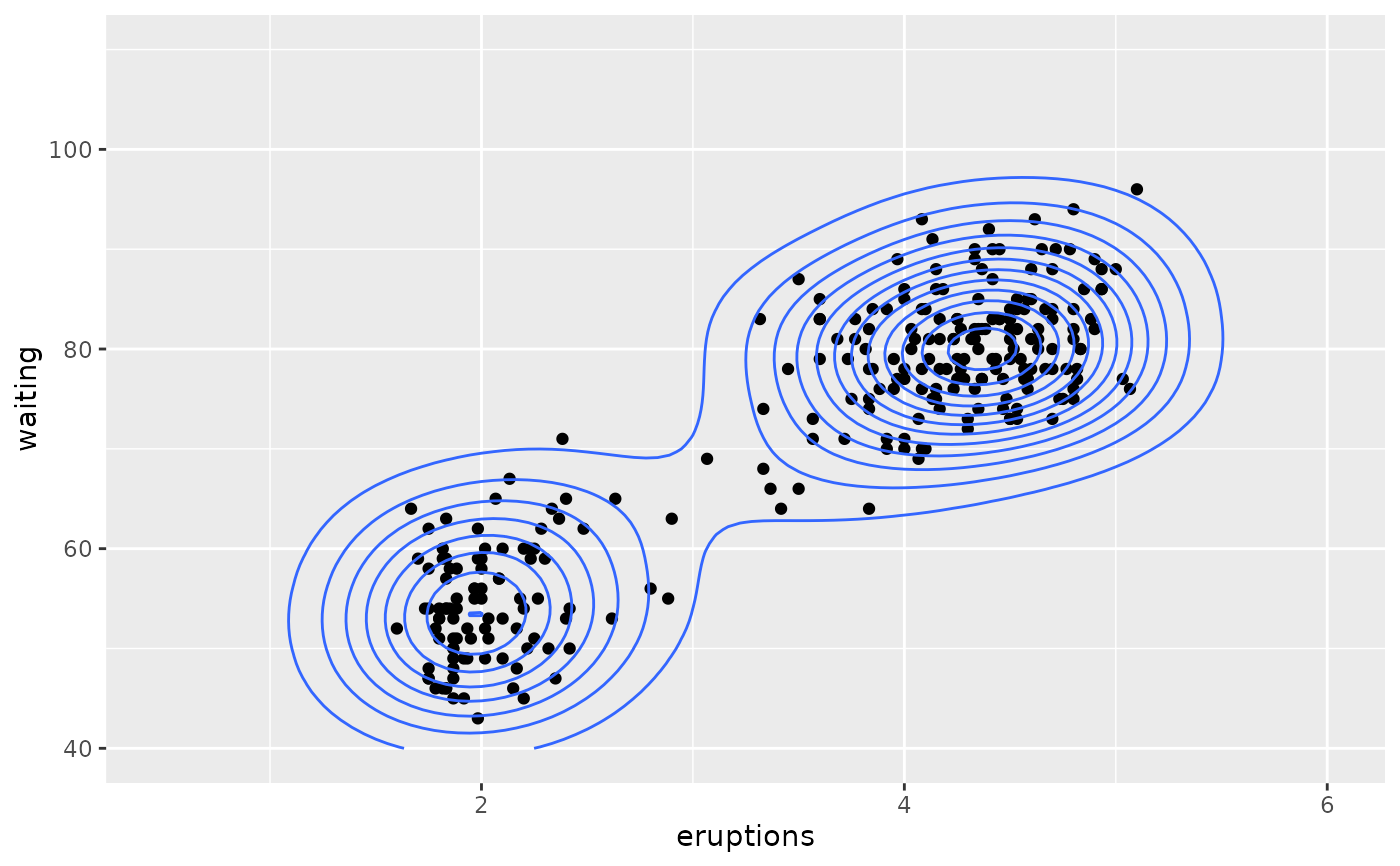# \donttest{
# contour bands
m + geom_density_2d_filled(alpha = 0.5)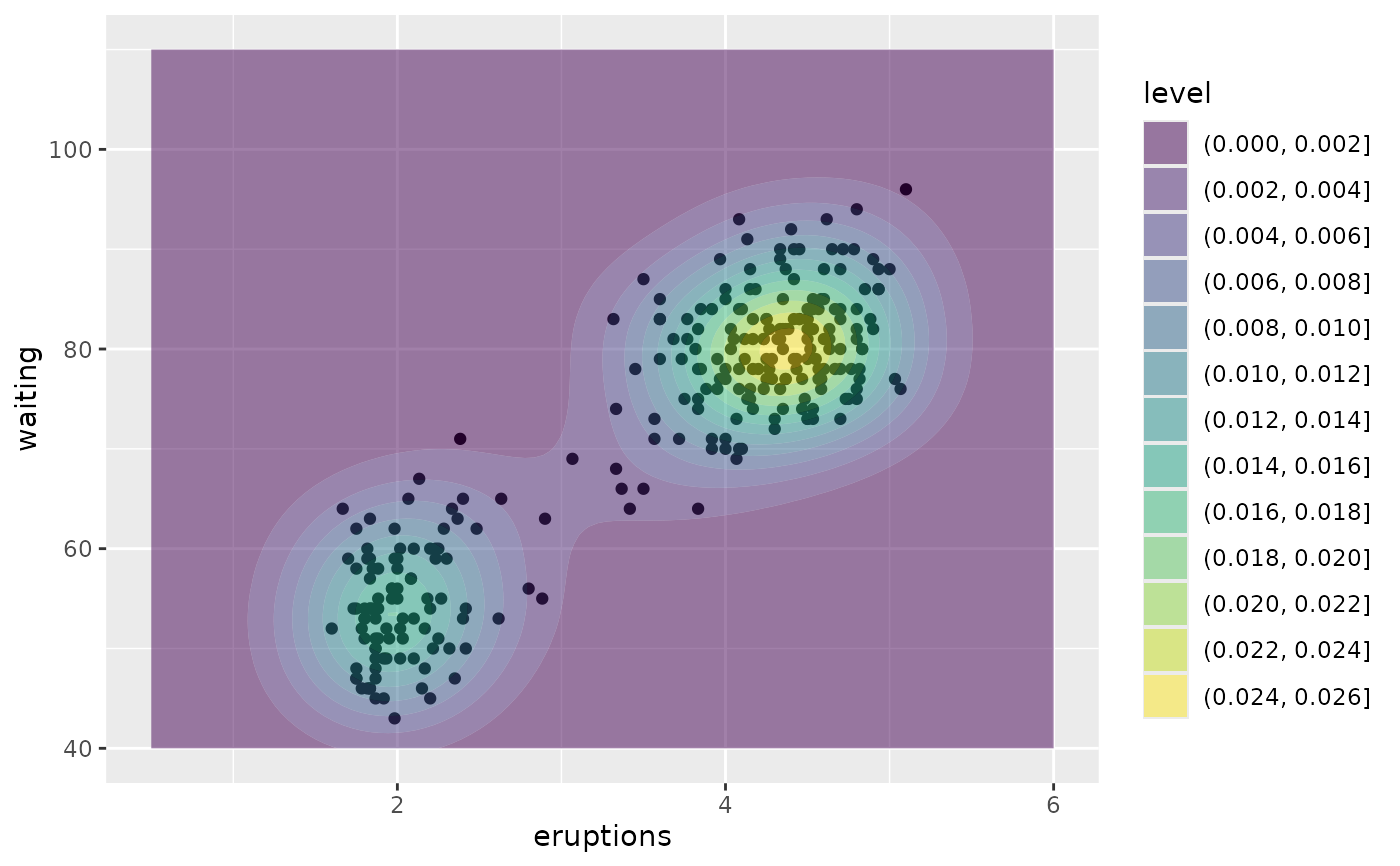# contour bands and contour lines
m + geom_density_2d_filled(alpha = 0.5) +
geom_density_2d(size = 0.25, colour = "black")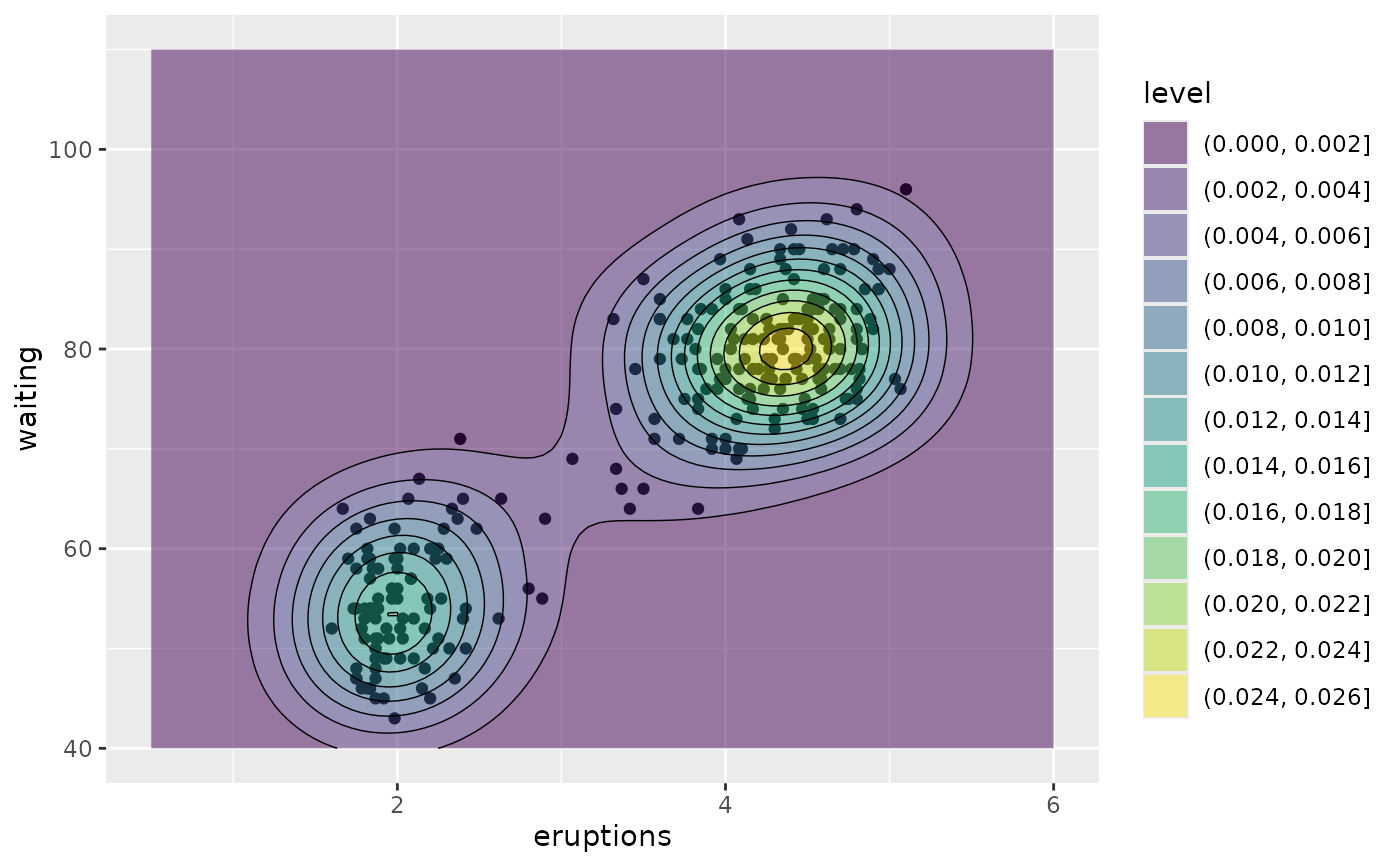set.seed(4393)
dsmall <- diamonds[sample(nrow(diamonds), 1000), ]
d <- ggplot(dsmall, aes(x, y))
# If you map an aesthetic to a categorical variable, you will get a
# set of contours for each value of that variable
d + geom_density_2d(aes(colour = cut))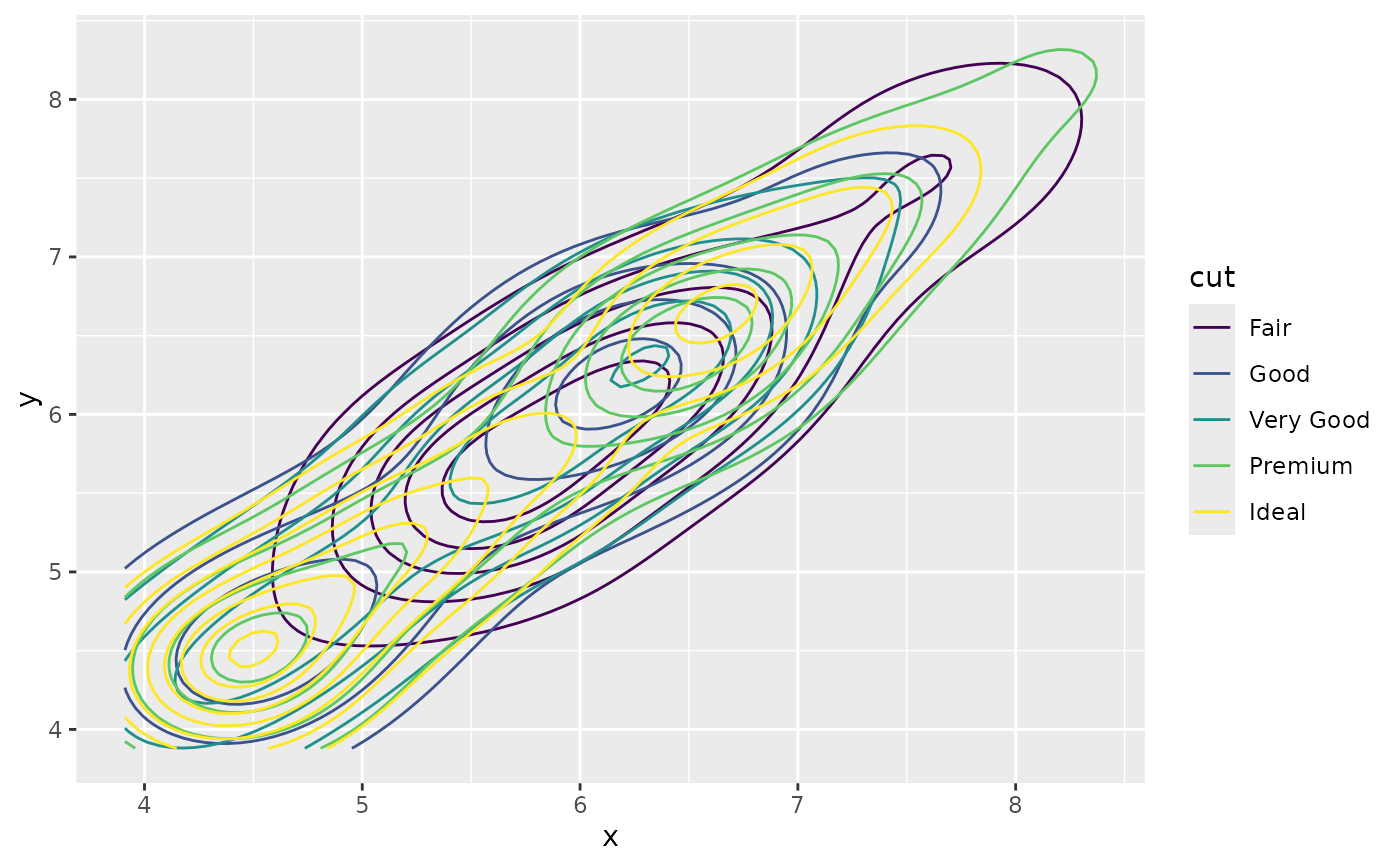# If you draw filled contours across multiple facets, the same bins are
# used across all facets
d + geom_density_2d_filled() + facet_wrap(vars(cut))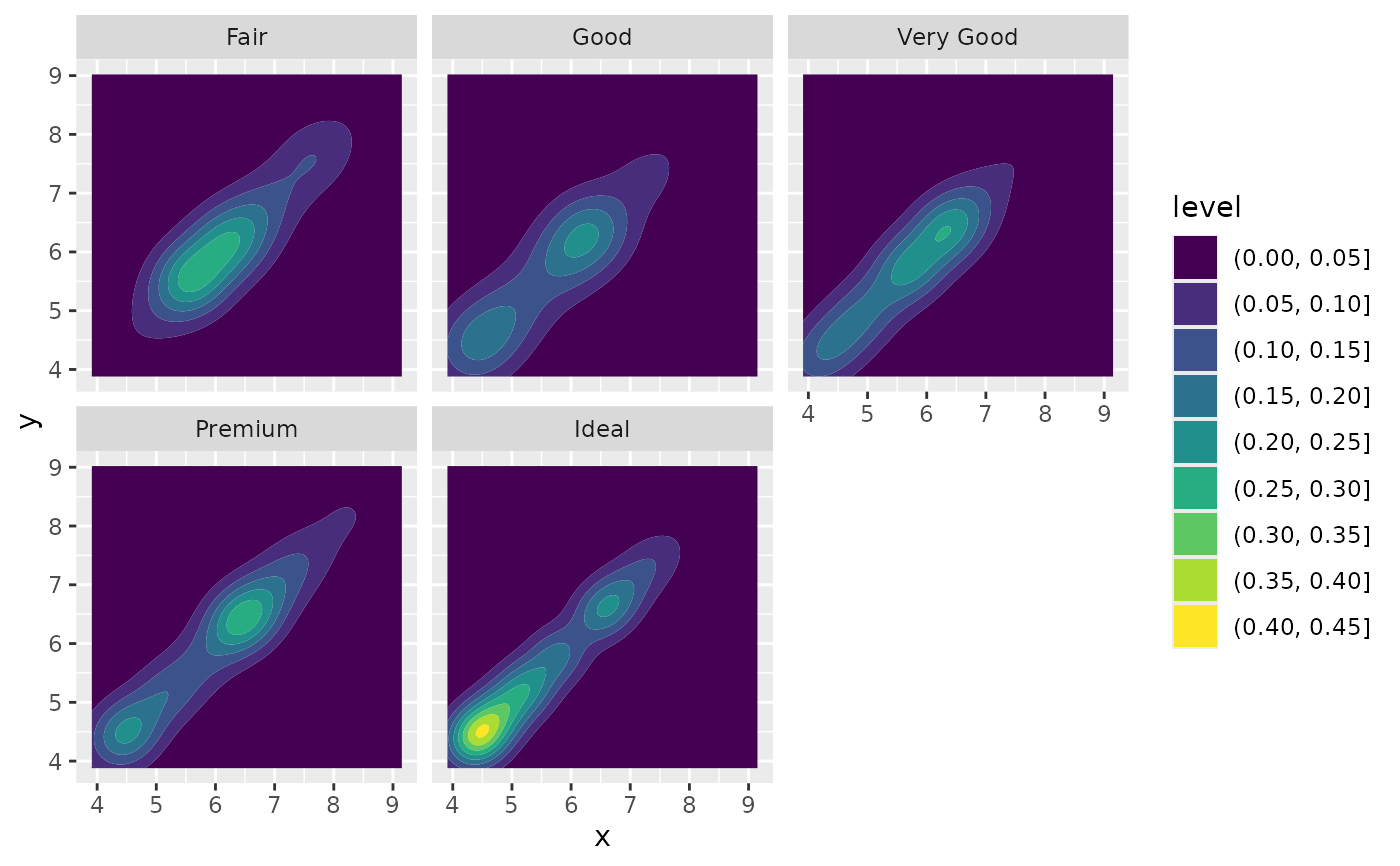# If you want to make sure the peak intensity is the same in each facet,
# use contour_var = "ndensity".
d + geom_density_2d_filled(contour_var = "ndensity") + facet_wrap(vars(cut))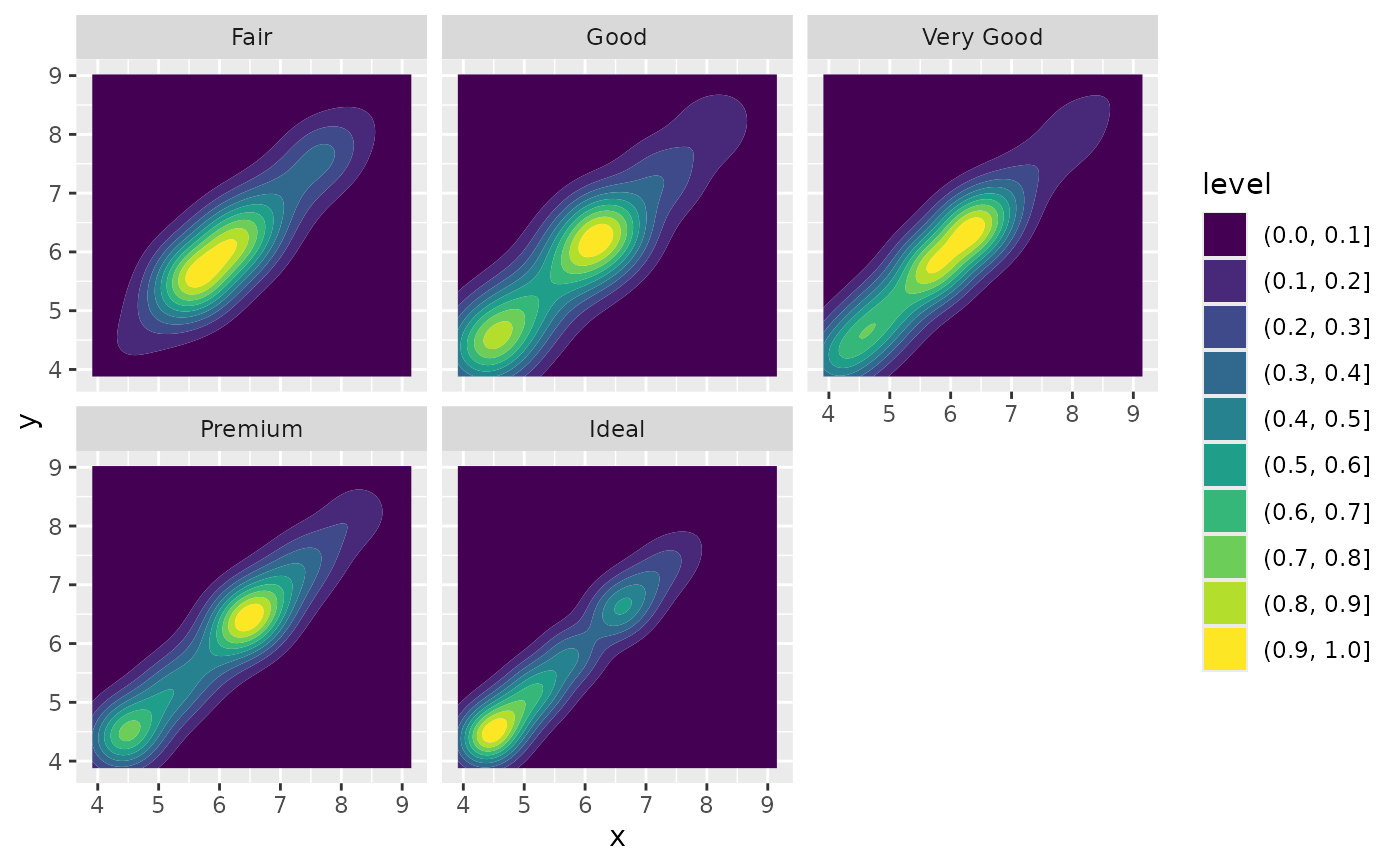# If you want to scale intensity by the number of observations in each group,
# use contour_var = "count".
d + geom_density_2d_filled(contour_var = "count") + facet_wrap(vars(cut))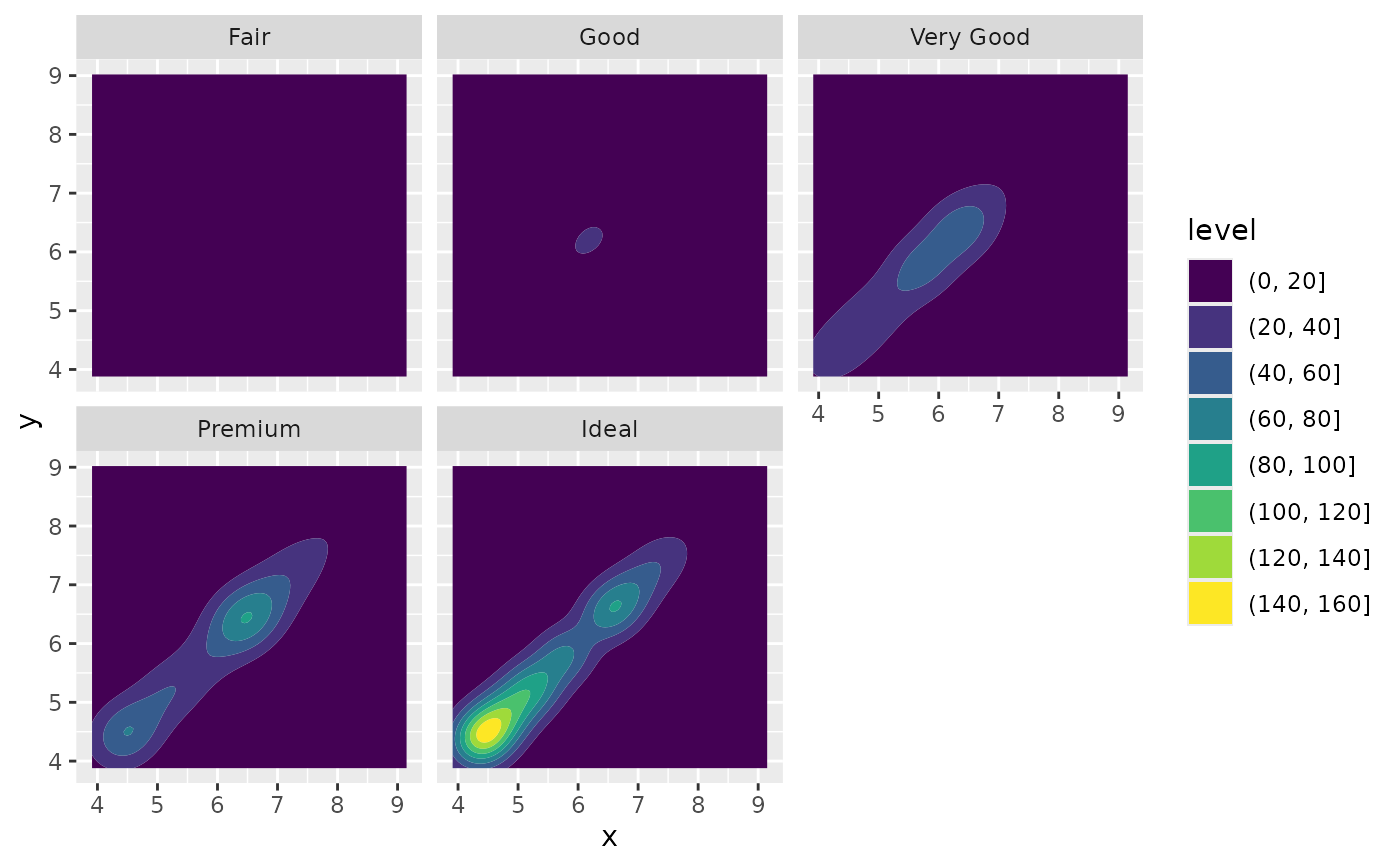# If we turn contouring off, we can use other geoms, such as tiles:
d + stat_density_2d(
geom = "raster",
aes(fill = after_stat(density)),
contour = FALSE
) + scale_fill_viridis_c()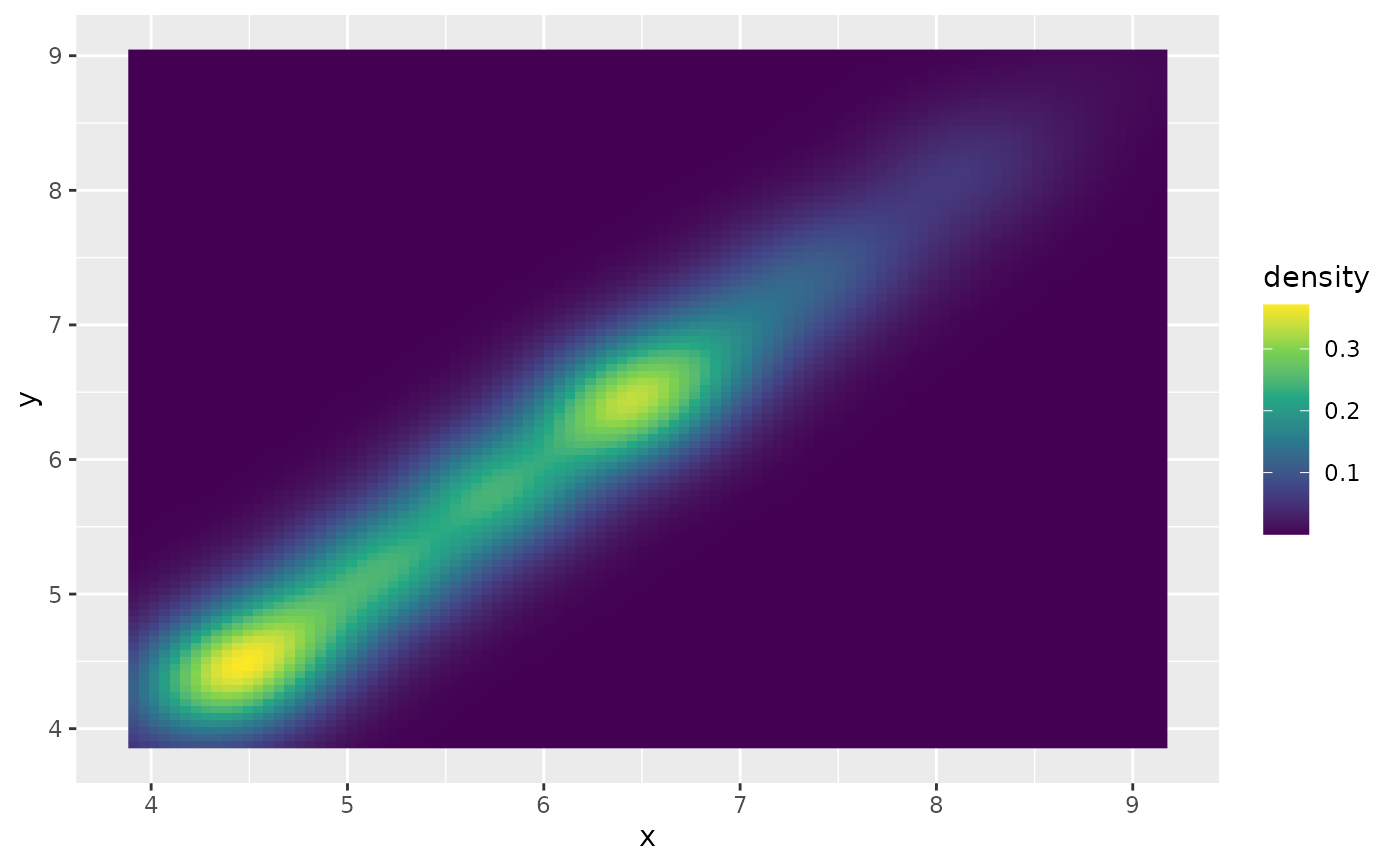# Or points:
d + stat_density_2d(geom = "point", aes(size = after_stat(density)), n = 20, contour = FALSE)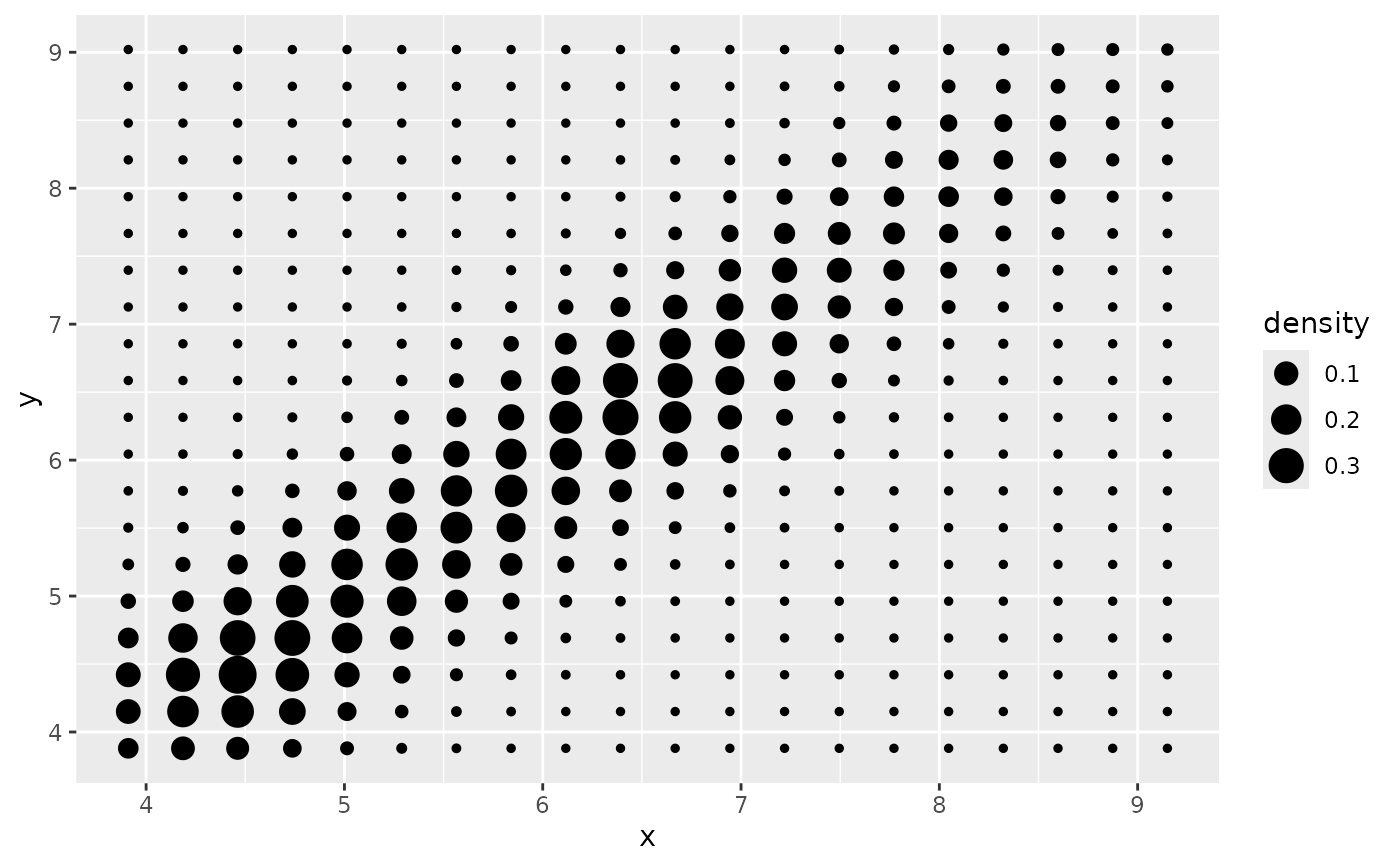# }